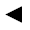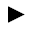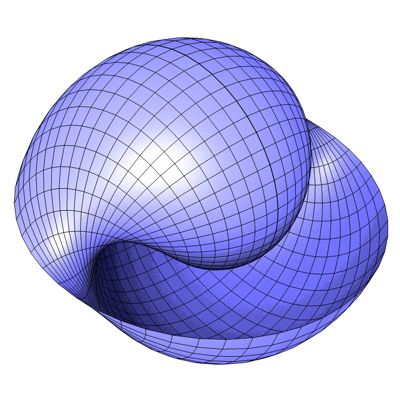﻿﻿﻿ math handbook calculator - Fractional Calculus Computer Algebra System software+++=﻿[back]

## 3.34. Snail Surface

[next]The snail surface [ 8 ] is represented by the following equations.

 x = u cos(v) sin(u) 3-117 y = u cos(u) cos(v) 3-118 z = -u sin(v) 3-119

To represent the area, the two parameters u and v can assume the following values ​​(domain of definition), for example.

 u is an element from the set of numbers [0, 2 pi] v is an element from the number set [-pi, pi]

The domain of definition can be changed in the plugin.Fig. 38